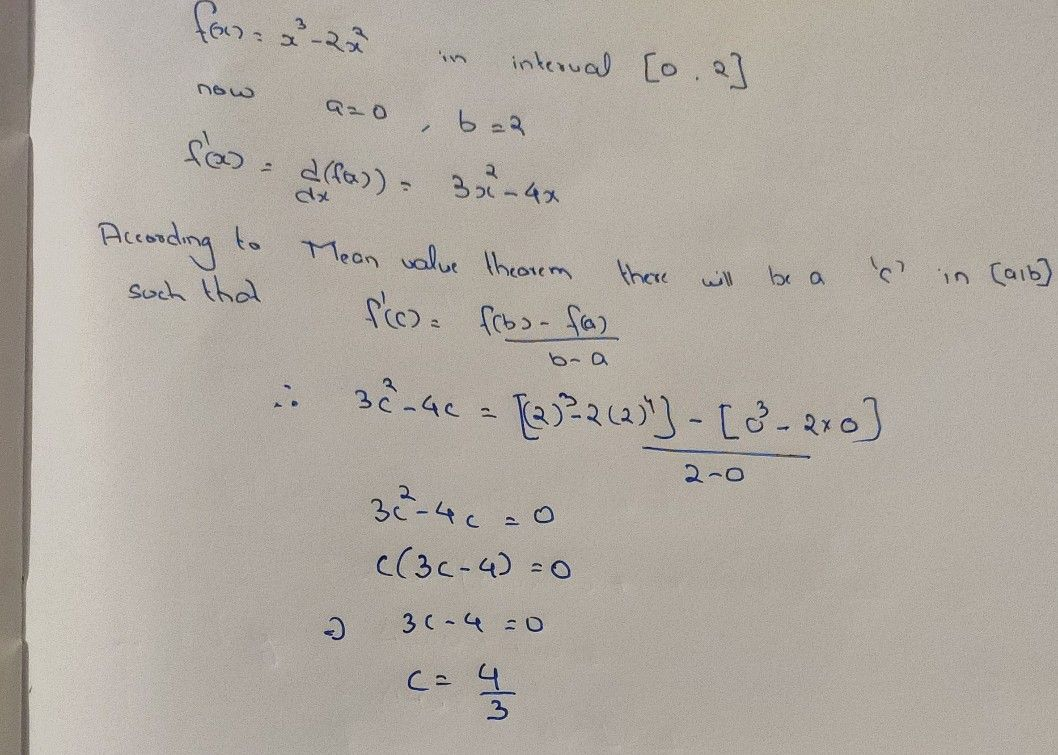Symbol
Problem$1$ Ifc is the number that satisfies the conclusion of the Mean Value Theorem for $f\left(x\right)=x^{3}-2x^{2}$ on the interval $0\leq x\leq 2$ then $c=$ $=$ $\left(A\right)0$ $\left(B\right)$ $\dfrac {1} {2}$ $\left(C\right)$ $1$ (D); $\dfrac {4} {3}$ (E) $2$
10th-13th grade
Calculus
SolutionQanda teacher - Aditya